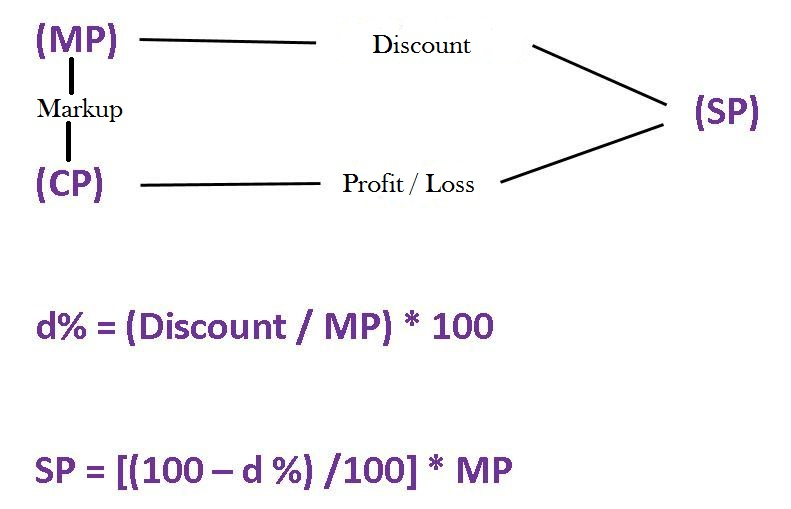# Quantitative Aptitude Notes : Profit & Loss

Profit & Loss is one of the very important topics in quantitative aptitude section in various banking exams. In this blog post, we will cover the basic definitions, important relations and short-cuts for this topic. So, read along!

###### IBPS PO 2017 Quantitative Aptitude Profit & Loss : Basic Definitions
• Cost Price ( CP ) : Price at which the seller brought the product.
• Selling Price ( SP ) : Price at which the seller sells the product to you or the customer.
• Marked Price ( MP ) : It is the price displayed on the product. ( Also, called MRP )
• Profit ( P ) : When SP > CP, seller makes a profit.

P = SP – CP ; P% = ( P / CP ) x 100

• Loss ( L ) : When SP < CP, seller suffers a loss.

L = CP – SP ; L% = ( P / CP ) x 100

Remember that both profit and loss are always calculated on the cost price of the product.

• Discounts ( D ) : The concession given by the seller on the marked price ( MP ) of the product is called discount.###### IBPS PO 2017 Quantitative Aptitude Profit & Loss : Important Relationship

All the terms mentioned in the above section are related to each other in very specific ways. The following representation will help you understand these connections and thus resolve all your confusions.SP = MP – Discount

Markup = MP – CP ; Markup % =  [ ( MP – CP ) / CP ] x 100

Remember that discount percentages are always calculated on the Marked Price ( MP ) of the product.

###### IBPS PO 2017 Quantitative Aptitude Profit & Loss : Multiplication Factor

The main formula for this is as follows :

Initial Value ( CP ) x Multiplication Factor ( MF ) = Final Value ( SP )###### IBPS PO 2017 Quantitative Aptitude Profit & Loss : Special Cases ( Short Cuts )

• For two successive profits or losses on an item, the formula for the resultant profit / loss % is :-

x + y + ( xy / 100)

Note: Both ‘x’ and ‘y’, here are profit / loss percentages, as given in the question. For profit use ‘+’ sign and for loss use ‘–’ sign. If result is in + then, there is an overall profit, if it is in ‘–’ then  there is an overall loss.

For more than two successive profits or losses on an item, the above formula for resultant profit / loss % is applies iterative-ly.

• If two items, which have the same CP, are sold such that one is at a profit of x% whereas the other is at a loss of x%. Then in the entire transaction, the seller neither suffers a loss nor receives profit.
• However, if two items with the same SP, are sold such that one is at a profit of x% whereas the other is at a loss of x%. Then in the entire transaction, the seller suffers a loss, which is given by the following formula :-

Overall Loss% = x^2 / 100        [ square of the common profit or loss % divided by 100 ]

All the best!!